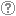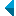# Iskanje po repozitorijuIskalni niz: išči po NaslovAvtorOpisKljučne besedeCelotno besediloLeto izida INALIIN NE išči po NaslovAvtorOpisKljučne besedeCelotno besediloLeto izida INALIIN NE išči po NaslovAvtorOpisKljučne besedeCelotno besediloLeto izida INALIIN NE išči po NaslovAvtorOpisKljučne besedeCelotno besediloLeto izida Vrsta gradiva: Vse vrste gradiv Habilitacijsko delo (m4) Višješolska diplomska naloga (m6) Specialistično delo (m3) Diplomsko delo * (dip) Magistrsko delo * (mag) Doktorska disertacija * (dok) Raziskovalni podatki ali korpus (data) * po starem in bolonjskem študiju Jezik: Vsi jezikiSlovenski jezikAngleški jezikNemški jezikHrvaški jezikSrbski jezikAfrikanski jezikAlbanski jezikArabski jezikArmenski jezikAzerbajdžanski jezikBolgarski jezikBosanski jezikČeški jezikČrnogorski jezikDanski jezikEstonski jezikFinski jezikFrancoski jezikGrški jezikGruzinski jezikIrski jezikItalijanski jezikJaponski jezikKatalonski jezikKazaški jezikKitajski jezikKorejski jezikLatinski jezikLatvijski jezikLitvanski jezikMadžarski jezikMakedonski jezikMalteški jezikNemški jezik (Avstrija)Nizozemski jezikNorveški jezikPoljski jezikPortugalski jezikRomunski jezikRuski jezikSlovanski jezik (ostali)Slovaški jezikSrbski jezik (cirilica)Španski jezikŠvedski jezikTurški jezikUkrajinski jezikNi določenVečjezičniNeznan jezikOstali Išči po: RUP    FAMNIT - Fakulteta za matematiko, naravoslovje in informacijske tehnologije    FHŠ - Fakulteta za humanistične študije    FM - Fakulteta za management    FTŠ Turistica - Fakulteta za turistične študije - Turistica    FVZ - Fakulteta za vede o zdravju    IAM - Inštitut Andrej Marušič    PEF - Pedagoška fakulteta    UPR - Univerza na Primorskem    ZUP - Založba Univerze na PrimorskemCOBISS    Univerza na Primorskem, Univerzitetna knjižnica - vsi oddelki Opcije: Prikaži samo zadetke s celotnim besedilom Ponastavi

 1 - 10 / 12121.On C[sup]2 rational motions of degree sixKarla Ferjančič, Marjetka Knez, Vito Vitrih, 2021, izvirni znanstveni članekKljučne besede: motion design, interpolation, rational spline motion, ▫\$C^2\$▫ continuityObjavljeno v RUP: 18.01.2021; Ogledov: 801; Prenosov: 34Povezava na celotno besedilo 2.Three-pencil lattice on triangulationsGašper Jaklič, Jernej Kozak, Marjetka Knez, Vito Vitrih, Emil Žagar, 2007, objavljeni znanstveni prispevek na konferenciOpis: In this paper, three-pencil lattices on triangulations are studied. The explicit representation of a lattice, based upon barycentric coordinates, enables us to construct lattice points in a simple and numerically stable way. Further, this representation carries over to triangulations in a natural way. The construction is based upon group action of S 3 on triangle vertices, and it is shown that the number of degrees of freedom is equal to the number of vertices of the triangulation.Ključne besede: numerical analysis, lattice, barycentric coordinates, triangulations, interpolationObjavljeno v RUP: 03.04.2017; Ogledov: 1855; Prenosov: 82Povezava na celotno besedilo 3.Barycentric coordinates for Lagrange interpolation over lattices on a simplexGašper Jaklič, Jernej Kozak, Marjetka Knez, Vito Vitrih, Emil Žagar, 2008, objavljeni znanstveni prispevek na konferenciOpis: In this paper, a ▫\$(d+1)\$▫-pencil lattice on a simplex in ▫\${\mathbb{R}}^d\$▫ is studied. The lattice points are explicitly given in barycentric coordinates. This enables the construction and the efficient evaluation of the Lagrange interpolating polynomial over a lattice on a simplex. Also, the barycentric representation, based on shape parameters, turns out to be appropriate for the lattice extension from a simplex to a simplicial partition.Ključne besede: numerical analysis, lattice, barycentric coordinates, simplex, interpolationObjavljeno v RUP: 03.04.2017; Ogledov: 1976; Prenosov: 136Povezava na celotno besedilo 4.On geometric Lagrange interpolation by quadratic parametric patchesGašper Jaklič, Jernej Kozak, Marjetka Knez, Vito Vitrih, Emil Žagar, 2008, izvirni znanstveni članekOpis: In the paper, the geometric Lagrange interpolation by quadratic parametric patches is considered. The freedom of parameterization is used to raise the number of interpolated points from the usual 6 up to 10, i.e., the number of points commonly interpolated by a cubic patch. At least asymptotically, the existence of a quadratic geometric interpolant is confirmed for data taken on a parametric surface with locally nonzero Gaussian curvature and interpolation points based upon a three-pencil lattice. Also, the asymptotic approximation order 4 is established.Ključne besede: numerična analiza, interpolacija, aproksimacija, parametrična ploskev, numerical analysis, interpolation, approximation, parametric surfaceObjavljeno v RUP: 03.04.2017; Ogledov: 2009; Prenosov: 136Povezava na celotno besedilo 5.Geometric Lagrange interpolation by planar cubic Pythagorean-hodograph curvesGašper Jaklič, Jernej Kozak, Marjetka Knez, Vito Vitrih, Emil Žagar, 2008, izvirni znanstveni članekOpis: In this paper, the geometric Lagrange interpolation of four points by planar cubic Pythagorean-hodograph (PH) curves is studied. It is shown that such an interpolatory curve exists provided that the data polygon, formed by the interpolation points, is convex, and satisfies an additional restriction on its angles. The approximation order is \$4\$. This gives rise to a conjecture that a PH curve of degree ▫\$n\$▫ can, under some natural restrictions on data points, interpolate up to ▫\$n+1\$▫ points.Ključne besede: numerical analysis, planar curve, PH curve, geometric interpolation, Lagrange interpolationObjavljeno v RUP: 03.04.2017; Ogledov: 1882; Prenosov: 129Povezava na celotno besedilo 6.An approach to geometric interpolation by Pythagorean-hodograph curvesGašper Jaklič, Jernej Kozak, Marjetka Knez, Vito Vitrih, Emil Žagar, 2012, izvirni znanstveni članekOpis: The problem of geometric interpolation by Pythagorean-hodograph (PH) curves of general degree ▫\$n\$▫ is studied independently of the dimension ▫\$d \ge 2\$▫. In contrast to classical approaches, where special structures that depend on the dimension are considered (complex numbers, quaternions, etc.), the basic algebraic definition of a PH property together with geometric interpolation conditions is used. The analysis of the resulting system of nonlinear equations exploits techniques such as the cylindrical algebraic decomposition and relies heavily on a computer algebra system. The nonlinear equations are written entirely in terms of geometric data parameters and are independent of the dimension. The analysis of the boundary regions, construction of solutions for particular data and homotopy theory are used to establish the existence and (in some cases) the number of admissible solutions. The general approach is applied to the cubic Hermite and Lagrange type of interpolation. Some known results are extended and numerical examples provided.Ključne besede: mathematics, parametric curve, PH curve, geometric interpolation, Lagrange interpolation, Hermite interpolation, cubic curves, homotopyObjavljeno v RUP: 03.04.2017; Ogledov: 1935; Prenosov: 69Povezava na celotno besedilo 7.Lagrange geometric interpolation by rational spatial cubic Bézier curvesGašper Jaklič, Jernej Kozak, Vito Vitrih, Emil Žagar, 2012, izvirni znanstveni članekOpis: V članku obravnavamo Lagrangeovo geometrijsko interpolacijo s prostorskimi racionalnimi kubičnimi Bézierovimi krivuljami. Pokažemo, da pod določenimi naravnimi omejitvami obstaja enolična rešitev problema. še več, rešitev je podana v preprosti zaključeni obliki in je zato zanimiva za praktične aplikacije. Asimptotična analiza potrdi pričakovani red aproksimacije, namreč 6. Numerični primeri nakažejo možnost uporabe te metode pri obetavni geometrijski nelinearni subdivizijski shemi.Ključne besede: numerična analiza, geometrijska Lagrageova interpolacija, racionalna Bézierova krivulja, prostorska krivulja, asimptotična analiza, subdivizija, numerical analysis, geometric Lagrange interpolation, rational Bézier curve, spatial curve, asymptotic analysis, subdivisionObjavljeno v RUP: 03.04.2017; Ogledov: 2118; Prenosov: 84Povezava na celotno besedilo 8.C [sup] 1 Hermite interpolation with spatial Pythagorean-hodograph cubic biarcsBohumír Bastl, Michal Bizzarri, Marjetka Knez, Miroslav Lávička, Kristýna Michálkova, Zbiněk Šír, Vito Vitrih, Emil Žagar, 2014, izvirni znanstveni članekOpis: In this paper the ▫\$C^1\$▫ Hermite interpolation problem by spatial Pythagorean-hodograph cubic biarcs is presented and a general algorithm to construct such interpolants is described. Each PH cubic segment interpolates ▫\$C^1\$▫ data at one point and they are then joined together with a ▫\$C^1\$▫ continuity at some unknown common point sharing some unknown tangent vector. Biarcs are expressed in a closed form with three shape parameters. Two of them are selected based on asymptotic approximation order, while the remaining one can be computed by minimizing the length of the biarc or by minimizing the elastic bending energy. The final interpolating spline curve is globally ▫\$C^1\$▫ continuous, it can be constructed locally and it exists for arbitrary Hermite data configurations.Ključne besede: mathematics, parametric curve, PH curve, Pythagorean-hodograph, Hermite interpolation, biarc, cubic curveObjavljeno v RUP: 03.04.2017; Ogledov: 1842; Prenosov: 37Povezava na celotno besedilo 9.Hermite interpolation by rational G [sup] k motions of low degreeGašper Jaklič, Bert Jüttler, Marjetka Knez, Vito Vitrih, Emil Žagar, 2013, izvirni znanstveni članekOpis: Interpolation by rational spline motions is an important issue in robotics and related fields. In this paper a new approach to rational spline motion design is described by using techniques of geometric interpolation. This enables us to reduce the discrepancy in the number of degrees of freedom of the trajectory of the origin and of the rotational part of the motion. A general approach to geometric interpolation by rational spline motions is presented and two particularly important cases are analyzed, i.e., geometric continuous quartic rational motions and second order geometrically continuous rational spline motions of degree six. In both cases sufficient conditions on the given Hermite data are found which guarantee the uniqueness of the solution. If the given data do not fulfill the solvability conditions, a method to perturb them slightly is described. Numerical examples are presented which confirm the theoretical results and provide an evidence that the obtained motions have nice shapes.Ključne besede: mathematics, numerical analysis, motion design, geometric interpolation, rational spline motion, geometric continuityObjavljeno v RUP: 03.04.2017; Ogledov: 1885; Prenosov: 36Povezava na celotno besedilo 10.Pythagorean-hodograph cycloidal curvesJernej Kozak, Marjetka Knez, Mladen Rogina, Vito Vitrih, 2015, izvirni znanstveni članekOpis: In the paper, Pythagorean-hodograph cycloidal curves as an extension of PH cubics are introduced. Their properties are examined and a constructive geometric characterization is established. Further, PHC curves are applied in the Hermite interpolation, with closed form solutions been determined. The asymptotic approximation order analysis carried out indicates clearly which interpolatory curve solution should be selected in practice. This makes the curves introduced here a useful practical tool, in particular in algorithms that guide CNC machines.Ključne besede: pitagorejski hodograf, C-krivulje, trigonometrične funkcije, karakterizacija, Hermiteova interpolacija, asimptotični red aproksimacije, Pythagorean-hodograph, C-curves, trigonometric functions, characterization, Hermite interpolation, asymptotic approximation orderObjavljeno v RUP: 08.08.2016; Ogledov: 2429; Prenosov: 196Povezava na celotno besedilo
Iskanje izvedeno v 0.05 sek.
Na vrh